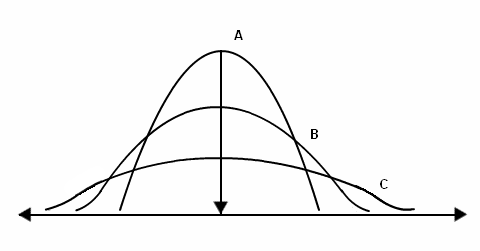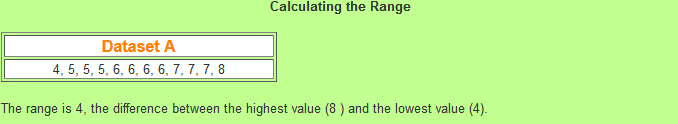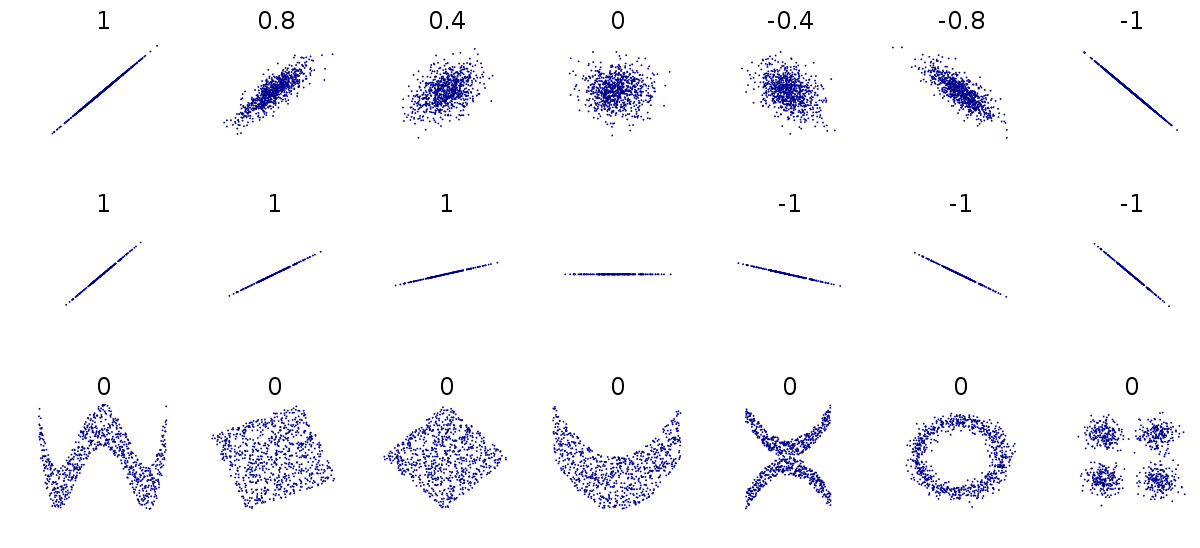medium.com Go back Open original

# The Guide to Rigorous Descriptive Statistics for Machine Learning and Data Science :

By Himanshu Sharma

Components of Uni-variate Analysis, Bi-variate Analysis and Multi-variate Analysis alongside Kurtosis, Skewness, Correlation explained mathematically and visually in a simple way.

A simple summary of what separates Inferential and Descriptive Statistics according to Wikipedia >

A descriptive statistic (in the count noun sense) is a summary statistic that quantitatively describes or summarizes features of a collection of information, while descriptive statistics in the mass noun sense is the process of using and analyzing those statistics. Descriptive statistics is distinguished from inferential statistics (or inductive statistics), in that descriptive statistics aims to summarize a sample, rather than use the data to learn about the population that the sample of data is thought to represent. This generally means that descriptive statistics, unlike inferential statistics, is not developed on the basis of probability theory, and are frequently nonparametric statistics. Even when a data analysis draws its main conclusions using inferential statistics, descriptive statistics are generally also presented. For example, in papers reporting on human subjects, typically a table is included giving the overall sample size, sample sizes in important subgroups (e.g., for each treatment or exposure group), and demographic or clinical characteristics such as the average age, the proportion of subjects of each sex, the proportion of subjects with related comorbidities, etc. [p1]

Therefore in layman terms, Descriptive Statistics is a form of representation of Data where we aim to summarize, label, organize and clean up the data given in various formats, but without drawing conclusions or inferences based off on the data we are using.

That is because we want to best describe data (Descriptive Statistics) and then feed it into our Machine Learning model making process through usage of features and sample sets, we don’t seek to infer the data ourselves (Inferential Statistics) since that is not our aim. That is before drawing conclusions from our data, we need to see how rich our data is in both quantity and quality by describing it as best as possible.

Descriptive Statistical Analysis includes two sub division of analysis methods depending of number of variables we have data on :>

Univariate analysis involves describing the distribution of a single variable, including its central tendency (including the mean, median, and mode) and dispersion (including the range and quartiles of the data-set, and measures of spread such as the variance and standard deviation). The shape of the distribution may also be described via indices such as skewness and kurtosis. Characteristics of a variable’s distribution may also be depicted in graphical or tabular format, including histograms and stem-and-leaf display. [p1]

These are methods to get a single value that somehow is central and a descriptor of the entire uni variate data set. There are three main measure’s of central tendency : Mean, Median and Mode.

Mean : Also known as average, it is simply all the values summed up divided by the number of values in our dataset.

Median : Median is the value separating the higher half from the lower half of the data, when arranged in ascending or descending order. For an odd numbered data sample, it is the central number whereas for an even numbered data sample, it is the average of the middle two values.

Mean Vs Median >

The main advantage of Median over Mean is that it is not affected by the presence of extremely large values or extremely small values (outliers) which may skew the mean. Median is a better reflection of a “typical” value of the set. Median can also be found using the Stem and Leaf Plot method.

Mode : The mode of a set of values is the term that appears most frequently thorough the sample, if there are two terms that appear the same number of times the data is bimodal, for three frequent values appearing same maximum number of times the data is trimodal, and for even more modes appearing in a data set, we call it Multimodal.

Central Tendency Measure’s are typical representations of a Frequency Distribution but they fail to give a complete picture of the distribution, they don’t tell us about the scatter-ness of the values within the distribution. For that we need Measure’s of Spread and Dispersion, which are a better indicator of variability within the dataset.Dispersion and Spread between A, B and C samples which aren’t reflected through Central Tendency Measure’s

The range is the difference between the smallest value and the largest value in a dataset. That is X(maximum)-X(minimum), therefore range is a big indicator to how vast the data spread is and how close the minimum and maximum values in the whole dataset are.Range for a sample dataset as : x(max) — x(min)

For above dataset, we have Range = 8–4 = 4

There is no universally accepted definition of a percentile. Although a satisfactory explanation cab help you understand what it means and how it can be used to rank data values. A percentile is not a percent and should not be confused with percentage; a percentile is a value (or the average of two values ) in the data set that marks a certain percentage of the way through the data. If you are at 80th percentile that means that 80% values were above you and 20% values are above yours.

For grouped percentiles we add up the all the percentages for the lower groups and add up half the percentages at the group we wish to calculate the percentile of.

Deciles are similar to Percentiles where they split up percentiles to better indicate rank of a value compared to the rest of the dataset values. The 1st decile is the 10th percentile (value dividing data that is 10% below it), 2nd decile is 20th percentile (value dividing data that is 20% below it)and so on …

Quartile is like decile but here we have Quarter splits of percentiles.

The first quartile, denoted Q1, is the value in the data set that holds 25% of the values below it. The third quartile, denoted Q3, is the value in the data set that holds 25% of the values above it.

Q1 -> 25 percentile (holds 25% values below it), median of lower half

Q2 -> 50 percentile (holds 50% values below it) also the median of whole data

Q3 -> 75 percentile (holds 75% values below it), median of upper half

We have IQR defined such that, Inter Quartile Range (IQR) = Q3-Q1 = 77–64 = 13 for the dataset in figure.

Both Variance and Standard Deviation are measures of the spread of data around the mean. Larger Standard Deviance and Variance values indicate a more dispersed dataset, while Zero Standard Deviance and Variance means all dataset values are the same.

Standard Deviation (σ) also called SD is a measure that is used to quantify the amount of variation or dispersion of a dataset. A smaller SD value indicates that more data values are closer to mean and larger SD values mean the dataset is widely dispersed. The standard deviation of a random variable, statistical population, data set, or probability distribution is the square root of its variance. It is algebraically simpler, though in practice less robust, than the average absolute deviation. A useful property of the standard deviation is that, unlike the variance, it is expressed in the same units as the data.

• Mathematically for non-grouped data, which is just basically a list of values, the standard deviation is given by :

Working out the mean for below data sample we have :

mean = (4 + 9 + 11 + 12 + 17 + 5 + 8 + 12 + 14) / 9 = 10.222

Summing up the above mean differences we get :

38.7 + 1.49 + 0.60 + 3.16 + 45.9 + 27.3 + 4.94 + 3.16 + 14.3 = 139.55

dividing above by n which is 9 we get 15.51 which is σ squared, now for σ :

we take square root of 15.51 which gives σ = 3.94

where :

• x mean = summation (f * x) / summation (f)

The following is an example dataset of grouped data, x given along with it’s frequency f :

For above we can calculate :

mean = (4x9 + 5x14 + 6x22 + 7x11 + 8x17) / (9 + 14 + 22 + 11 + 17)

Therefore mean = 451 / 73 = 6.178

summation of mean difference squared :

= 9*(-2.178)² + 14*(-1.178)² + 22*(-0.178)² + 11*(0.822)² + 17*(1.822)²

root (summation mean diff sq / summation f) = 126.685 / 73 = 1.784 = 1.33

So standard deviation for above sample is : 1.33

Also it is to be noted that Standard Deviation has two form based off on the population size of the sample data and whether you want to generalize it or not:

• Sample Standard Deviation :

Usually where we don’t have complete Population sample data, Sample Standard Deviation is used and we generalize our findings for the entire population, mathematically >

• Population Standard Deviation :

In cases where complete Population Sample data is present, Population standard deviation is used, here there is no need to generalize the result and data samples can be further used to find Sample Standard Deviation for specific population ranges, mathematically we use>

Variance (σ squared) measures how far a dataset is spread out. The technical definition is “The average of the squared differences from the mean”. We use variance to see how individual numbers relate to each other within a data set, rather than using broader mathematical techniques such as arranging numbers into Quartiles. A drawback to variance is that it gives added weight to numbers far from the mean (outliers), since squaring these numbers can skew interpretations of the data. The advantage of variance is that it treats all deviations from the mean the same regardless of direction- as a result, the squared deviations cannot sum to zero and give the appearance of no variability at all in the data. The drawback of variance is that it is not easily interpreted, and the square root of its value is usually taken to get the standard deviation of the data set in question.

Mathematically :

• σ squared is variance
• χ is the value of an individual data point
• μ is the mean of data points
• N is the total # of data points

The variance for a population (a parameter not a statistic)is calculated by:

1. Finding the mean (the average).
2. Subtracting the mean from each number in the data set and then squaring the result. The results are squared to make the negatives positive. Otherwise negative numbers would cancel out the positives in the next step. It’s the distance from the mean that’s important, not positive or negative numbers.
3. Averaging the squared differences.

It is the degree of distortion of Normal Distribution also called ‘tapering’ of data distribution, Skewness is a measure of symmetry or more precisely lack of symmetry in our Data Distribution. A distribution, or data set, is symmetric if it looks the same to the left and right of the center point, and skewness is a measure of how asymmetric the distribution is.

1. negative skew: The left tail is longer; the mass of the distribution is concentrated on the right of the figure. It has a few relatively low values. The distribution is said to be left-skewed. In such a distribution, the mean is lower than median (or equivalently, the mean is lower than the mode; in which case the skewness is lower than zero.
2. positive skew: The right tail is longer; the mass of the distribution is concentrated on the left of the figure. It has a few relatively high values. The distribution is said to be right-skewed. In such a distribution, the mean is greater than median, or equivalently, the mean is greater than the mode; in which case the skewness is greater than zero.

In a skewed (unbalanced, lopsided) distribution, the mean is farther out in the long tail than is the median. If there is no skewness or the distribution is symmetric like the bell-shaped normal curve then the mean = median = mode.

Gauging -> Excessive Skewness or Moderate Skewness :

• For skewness between -0.5 and 0.5 our data is quite symmetrical and not skewed too much.
• For skewness between -1.0 and -.05 or 0.5 and 1.0 our data is moderately skewed.
• For skewness exceeding 1.0 or below -1.0 our data is excessively skewed.

We have many formulas that can be used to derive a Skew coefficient from data values instead of relying on visual plots to understand if it’s positive or negative. Some of them are :

• Fisher-Pearson coefficient of skewness :
• Galton skewness (also known as Bowley’s skewness) :

Kurtosis is a measure of outliers present in the distribution. It is a measure that indicates how much data is contained in the tails of your data. Kurtosis can also said to be a measure of the “tailedness” of the probability distribution of a real-valued random variable.

• positive value tells us that our dataset has heavy-tails. (lot of data in tails)
• negative value means that dataset has light-tails. (little data in tails)

Excess Kurtosis >

The excess Kurtosis is defined as Kurtosis minus 3, it is a measure of how the distribution’s tails compare to normal (see Aldrich, E, 2014). Three different Kurtosis possibilities are :

• Mesokurtic : Distribution with zero excess Kurtosis are called mesokurtic, or mesokurtotic, the most common example would be Normal Distribution and it’s family, as standard Normal Distribution has kurtosis of three.
• Leptokurtic (Kutosis > 3) : A distribution with positive excess kurtosis is called leptokurtic, or leptokurtotic. “Lepto-” means “slender”. In terms of shape, a leptokurtic distribution has fatter tails. Examples of leptokurtic distributions include the Student’s t-distribution, Rayleigh distribution, Laplace distribution, exponential distribution, Poisson distribution and the logistic distribution. Such distributions are sometimes termed super-Gaussian.
• Platykurtic (Kurtosis < 3): A distribution with negative excess kurtosis is called platykurtic, or platykurtotic. “Platy-” means “broad”. In terms of shape, a platykurtic distribution has thinner tails. Examples of platykurtic distributions include the continuous and discrete uniform distributions, and the raised cosine distribution. The most platykurtic distribution of all is the Bernoulli distribution with p = 1/2 (for example the number of times one obtains “heads” when flipping a coin once, a coin toss), for which the excess kurtosis is −2. Such distributions are sometimes termed sub-Gaussian.

When a sample consists of more than one variable, descriptive statistics may be used to describe the relationship between pairs of variables. In this case, descriptive statistics include:

The main reason for differentiating univariate and bivariate analysis is that bivariate analysis is not only simple descriptive analysis, but also it describes the relationship between two different variables. Quantitative measures of dependence include correlation (such as Pearson’s r when both variables are continuous, or Spearman’s rho if one or both are not) and covariance (which reflects the scale variables are measured on). The slope, in regression analysis, also reflects the relationship between variables. The unstandardized slope indicates the unit change in the criterion variable for a one unit change in the predictor. The standardized slope indicates this change in standardized (z-score) units. Highly skewed data are often transformed by taking logarithms. Use of logarithms makes graphs more symmetrical and look more similar to the normal distribution, making them easier to interpret intuitively.[p1]

Correlation is any statistical association (statistical relationship) between two random variables or bi-variate data. In common cases though, it refers to how close of a linear relationship two variables have with each other.

Some examples of data that have a high correlation:

• Hype for Machine Learning Vs people doing Machine Learning work.
• Distance you run each day and your fitness level.
• The amount of typing you do and your average typing wpm.

Some examples of data that have a low correlation (or none at all):

• How much TV you watch vs how much food your dog eats.
• Possible release dates for Avenger’s : Endgame (2019) and possible release dates for Joker (2019) [not too sure though]

Mathematically, it depends on correlation coefficientr’ it ranges from -1.0 to +1.0 and the closer r is to -1.0 or +1.0, the more closely related the two variables are. For correlation due to value being closer to -1.0, it is also called ‘inverse’ correlation.Several Sets of (x,y) points with Pearson Coefficient ‘r’ for each set.

Usually, in statistics, we measure four types of correlations: Pearson correlation, Kendall rank correlation, Spearman correlation, and the Point-Biserial correlation. But Pearson r correlation is the most widely used technique for linear relationship between variables :

There are five assumptions that are made with respect to Pearson’s correlation:

1. The variables must be either interval or ratio measurements.
2. The variables must be approximately normally distributed.
3. There is a linear relationship between the two variables.
4. Outliers are either kept to a minimum or are removed entirely. (This can be done by removing the extreme outliers after plotting the data)
5. There is homoscedasticity of the data. In statistics, a sequence or a vector of random variables is homoscedastic if all random variables in the sequence or vector have the same finite variance. This is also known as homogeneity of variance. [p2]
`If you found this useful and informative, please let me know by clapping or commenting ! Also for any queries you may have in regard to the above, ask me by commenting or tweeting @himanshuxd`